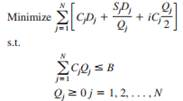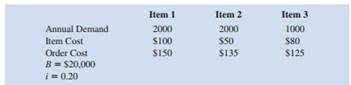### Create an Account

Home / Questions / The economic order quantity EOQ model is a classical model used for controlling inventory ...

# The economic order quantity EOQ model is a classical model used for controlling inventory and satisfying demand Costs included in the model are holding cost per unit ordering cost and the cost of

The economic order quantity (EOQ) model is a classical model used for controlling inventory and satisfying demand. Costs included in the model are holding cost per unit, ordering cost, and the cost of goods ordered. The assumptions for that model are that only a single item is considered, that the entire quantity ordered arrives at one time, that the demand for the item is constant over time, and that no shortages are allowed. Suppose we relax the first assumption and allow for multiple items that are independent except for a budget restriction. The following model describes this situation:

Let D= annual demand for item j

Cj = unit cost of item j

Sj = cost per order placed for item j

i = inventory carrying charge as a percentage of the cost per unit

B = the maximum amount of investment in goods

N = number of items

The decision variables are Qj , the amount of item j to order. The model is:In the objective function, the first term is the annual cost of goods, the second is the annual ordering cost (Dj /Qj is the number of orders), and the last term is the annual inventory holding cost (Qj /y2 is the average amount of inventory).

a. Set up a spreadsheet model and for the following data:b. Solve the problem using Excel Solver.

Apr 25 2020 View more View LessSubscribe To Get Solution